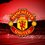# Help needed in an MCQ

$\large \displaystyle I_n \int_{\pi}^\pi \frac1{1 + 2^{\sin(x/2)} } \left( \frac{\sin(nx/2)}{\sin(x/2)} \right)^2 \, dx$

Hi brilliant! I've a doubt in calculus! The question is above.

The options are:

A) ${ I }_{ n }={ I }_{ n+1 }\forall n\ge 1$

B) ${ I }_{ 0 },{ I }_{ 1 },{ I }_{ 2 },{ ...,I }_{ n }\quad are\quad in\quad AP$

C) $\sum _{ m=0 }^{ 9 }{ { I }_{ 2m }=90\pi }$

D) $\sum _{ m=0 }^{ 10 }{ { I }_{ m }=55\pi }$

More than one options are right5 years, 8 months ago

This discussion board is a place to discuss our Daily Challenges and the math and science related to those challenges. Explanations are more than just a solution — they should explain the steps and thinking strategies that you used to obtain the solution. Comments should further the discussion of math and science.

When posting on Brilliant:

• Use the emojis to react to an explanation, whether you're congratulating a job well done , or just really confused .
• Ask specific questions about the challenge or the steps in somebody's explanation. Well-posed questions can add a lot to the discussion, but posting "I don't understand!" doesn't help anyone.
• Try to contribute something new to the discussion, whether it is an extension, generalization or other idea related to the challenge.

MarkdownAppears as
*italics* or _italics_ italics
**bold** or __bold__ bold
- bulleted- list
• bulleted
• list
1. numbered2. list
1. numbered
2. list
Note: you must add a full line of space before and after lists for them to show up correctly
paragraph 1paragraph 2

paragraph 1

paragraph 2

[example link](https://brilliant.org)example link
> This is a quote
This is a quote
    # I indented these lines
# 4 spaces, and now they show
# up as a code block.

print "hello world"
# I indented these lines
# 4 spaces, and now they show
# up as a code block.

print "hello world"
MathAppears as
Remember to wrap math in $$ ... $$ or $ ... $ to ensure proper formatting.
2 \times 3 $2 \times 3$
2^{34} $2^{34}$
a_{i-1} $a_{i-1}$
\frac{2}{3} $\frac{2}{3}$
\sqrt{2} $\sqrt{2}$
\sum_{i=1}^3 $\sum_{i=1}^3$
\sin \theta $\sin \theta$
\boxed{123} $\boxed{123}$

Sort by:

First substitute x by -x and add to get rid of the denominator. Then use the steps in the solution of this problem. This should help you.

- 5 years, 8 months ago

- 5 years, 8 months ago

First substitute x by -x and add to get rid of the denominator.And then evaluate $I_0$,$I_1$,$I_2$.... which will come out to be $0,\pi ,2\pi.....$ hence they will be in AP .So B,C,D.

And that problem by Sudeep can also be evaluated like that.

- 5 years, 7 months ago

Ooh nice :)

- 5 years, 7 months ago

i think you have written the statement wrong it should be -pi to pi , correct it then only answers can be given @Aditya Kumar if it's -pi to pi then the answer would be B,C,D .....just correct it !

- 4 years, 7 months ago

I'm sure that it's one of a, b, c or d.

- 5 years, 8 months ago

Thanks for enlightening!

- 5 years, 8 months ago

There is no one :(

- 5 years, 8 months ago

What????

- 5 years, 8 months ago